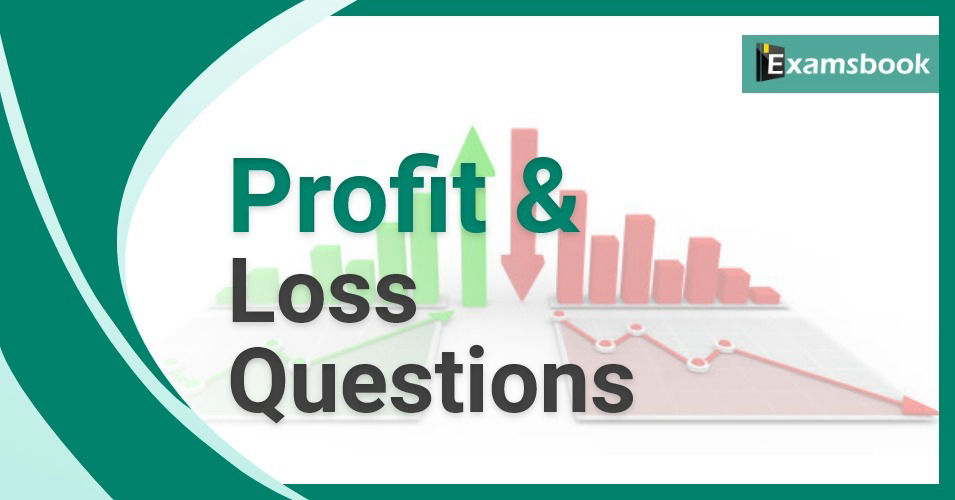• Save

# Profit and Loss Questions and Answers for SSC and Bank ExamsHello students, You can practice aptitude questions topic-wise with Examsbook. Today in this post you can practice with profit and loss questions and answers for SSC and Bank exams. Improve your performance in profit and loss questions and practice with important profit and loss questions and answers.

So those students who are preparing for SSC or bank exams can practice profit and loss problems with solutions. Often students make mistake to use profit and loss formulas. So, you should also learn to use profit and loss formulas with examples for your competitive exams. Below is the list of other topics of profit and loss.

You can read also: profit and loss questions and answers in Hindi.

## Profit and Loss Questions and Answers

Q.1 If by selling an article for Rs. 100 a man gains Rs. 15, then his gain percent is….

(A) 15%

(B) 12 2/3 %

(C) 17 11/17 %

(D) 17 ¼ %

Ans .  C

Q.2 When A commodity is sold for Rs. 34.80, there is a loss of 25%. What is the cost price of the commodity?

(A) Rs. 46.40

(B) Rs. 26.10

(C) Rs. 43

(D) Rs. 43.20

Ans .  A

To know more about Time and Speed: time-and-speed-objective-type-question-for-competitive-exams

Q.3 If the S.P. of an article for is 4/3 times its C.P.  the profit percent is…….

(A) 33 1/3%

(B) 25 1/4 %

(C) 20 ½ %

(D) 20 ¾ %

Ans .  A

Q.4 By selling an article for Rs. 19.50, a dealer makes a profit of 30%. By how much should he increase his S.P. so as to make a profit of 40%?

(A) Rs. 1.50

(B) Rs. 1.75

(C) Rs. 2

(D) Rs. 3

Ans .  A

Q.5 The C.P. of 20 articles is the same as S.P. of 15 articles. The profit percent is….

(A) 25%

(B) 30%

(C) 33 1/3 %

(C) 50%

Ans .  C

Q.6 A fruit seller purchases oranges at the rate of 3 for Rs. 5 and sells them at 2 for Rs. 4. His profit is…….

(A) 10%

(B) 11%

(C) 20%

(D) 25%

Ans .  C

Q.7 A dealer professing to sell his goods at C.P., uses a 900-gm weight for a kg. His gain percent is……

(A) 9%

(B) 10%

(C) 11%

(D) 11 1/9 %

Ans .  D

Q.8 A man buys eggs at 2 for Rs. 1 and an equal number at 3 for Rs. 2 and sells the whole at 5 for Rs. 3. His gain or loss percent is…….

(A) 2 2/7 %

(B) 3 6/7 %

(C) 3 2/7 %

(D) 2 6/7 %

Ans .  D

Q.9 A sells a bicycle to B at a profit of 20% and B sells it to C at a profit of 25%. If C pays Rs. 1500, what did A pay for it?

(A) Rs. 825

(B) Rs. 1000

(C) Rs.110

(D) Rs. 1125

Ans .  B

Q.10 Two mixers and a TV costs Rs. 7000, while 2 TVs and a mixer cost Rs. 9800. The values of one TV is……

(A) Rs. 2800

(B) Rs. 2100

(C) Rs. 4200

(D) Rs. 8400

Ans .  C